Gradarius is all about solving calculus problems and showing your work. You have a great deal of freedom when it comes to writing the solution to a problem, but it’s still important to understand the basic philosophy behind how solutions should be structured.

Solutions that you enter in Gradarius are structured in terms of cells and rows. A cell is the basic building block of any solution. It is a space in which to enter a mathematical  expression: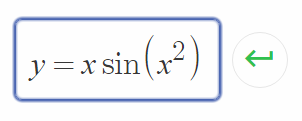After entering an expression in a cell, you can rewrite it in a new cell, whose content you can rewrite in yet another cell, and so on, thus yielding a sequence of cells each of which follows logically from  the one that came before it. Such a sequence of cells comprises a row: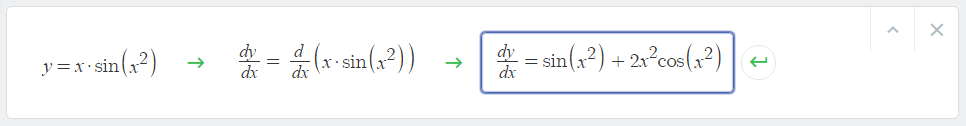You can enter different types of mathematical expressions in a given cell. You might enter a function, likebut you could also enter an equation, likeor a numerical expression likeNote, however, that the cells you create in a given row should always  contain expressions of the same type. It would be bad form, for instance, to rewrite the equation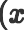-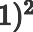as the number  1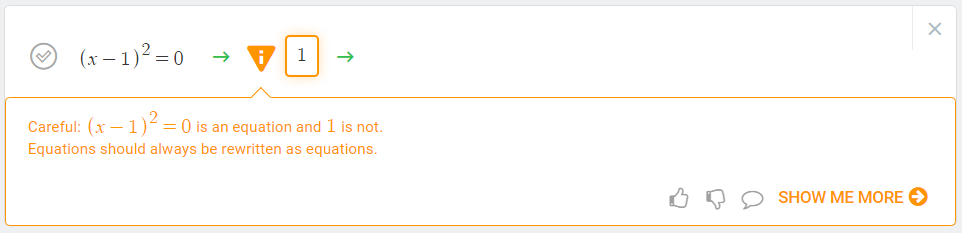It should instead be rewritten as the equation:Be sure to rewrite equations as equations, functions as functions, numbers as numbers, and so on!

Once you’re done rewriting an expression within a row, you can continue your work in a new row. Once you’ve entered the full solution to a problem, it remains only to select your final answer and submit your work for grading.

A solution, to recap, consists of a collection of rows, and each row consists of cells containing mathematical expressions of the same type.